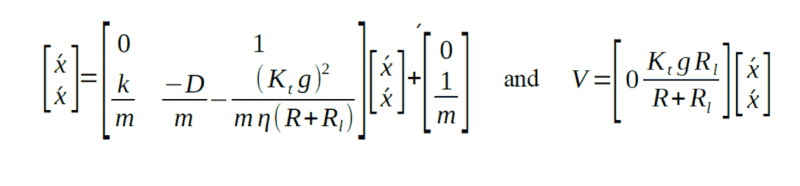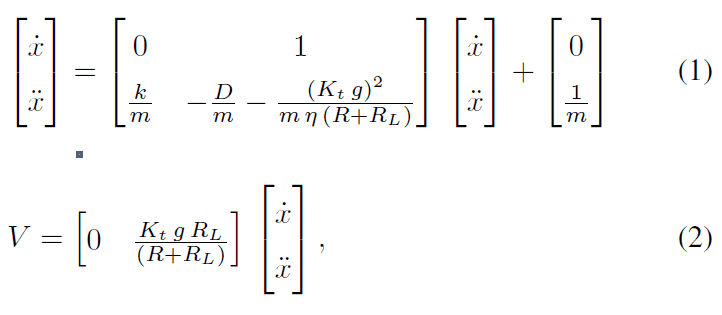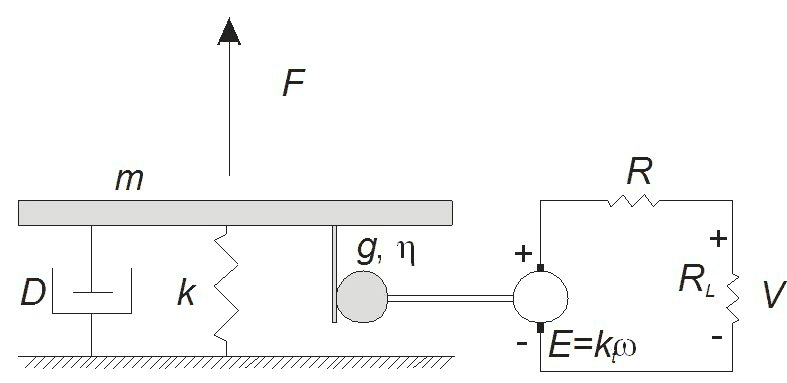# Vertical format and dotted / double dotted x

## Homework Statement

I am trying to understand what [x^. x^..] means, and information regarding the vertical format it is in .
Can someone please help me understand, or refer me to a website that can aid me. Thank you!

The system is given by

[PLAIN]https://www.physicsforums.com/file:///C:/Users/Guest/AppData/Local/Temp/msohtmlclip1/01/clip_image002.png [Broken] [Broken] and [PLAIN]https://www.physicsforums.com/file:///C:/Users/Guest/AppData/Local/Temp/msohtmlclip1/01/clip_image004.png [Broken] [Broken]

where x is the position and V the voltage across the load resistance.

K= 150000 N/m

D=10 Ns/m

Kt = 0.0728

Ƞ= 50%

R= 19.2 Ω

Rl = 150 Ω

m= my manipulated (independent) variable

## Homework Equations

[PLAIN]https://www.physicsforums.com/file:///C:/Users/Guest/AppData/Local/Temp/msohtmlclip1/01/clip_image002.png [Broken] [Broken] and [PLAIN]https://www.physicsforums.com/file:///C:/Users/Guest/AppData/Local/Temp/msohtmlclip1/01/clip_image004.png [Broken] [Broken]

## The Attempt at a Solution

I believe x^. and x^.. may refer to derivatives and the vertical format may have something to do with vector notation. I just need more specifics if I am to continue my investigation.

#### Attachments

• math.docx
15.7 KB · Views: 162
Last edited by a moderator:

haruspex
Homework Helper
Gold Member
Unfortunately the links to the images appear to be broken, and when viewing the .docx with OpenOffice the expressions appear blank.

SammyS
Staff Emeritus
Homework Helper
Gold Member

## Homework Statement

I am trying to understand what [x^. x^..] means, and information regarding the vertical format it is in .
Can someone please help me understand, or refer me to a website that can aid me. Thank you!

The system is given by

[PLAIN]https://www.physicsforums.com/file:///C:/Users/Guest/AppData/Local/Temp/msohtmlclip1/01/clip_image002.png [Broken] [Broken] and [PLAIN]https://www.physicsforums.com/file:///C:/Users/Guest/AppData/Local/Temp/msohtmlclip1/01/clip_image004.png [Broken] [Broken]

where x is the position and V the voltage across the load resistance.

K= 150000 N/m

D=10 Ns/m

Kt = 0.0728

Ƞ= 50%

R= 19.2 Ω

Rl = 150 Ω

m= my manipulated (independent) variable

## Homework Equations

[PLAIN]https://www.physicsforums.com/file:///C:/Users/Guest/AppData/Local/Temp/msohtmlclip1/01/clip_image002.png [Broken] [Broken] and [PLAIN]https://www.physicsforums.com/file:///C:/Users/Guest/AppData/Local/Temp/msohtmlclip1/01/clip_image004.png [Broken] [Broken]

## The Attempt at a Solution

I believe x^. and x^.. may refer to derivatives and the vertical format may have something to do with vector notation. I just need more specifics if I am to continue my investigation.
Here is the image of the expressions you're wondering about.From your post I gather that the x with the grave' in the vector should be ## \dot{x} ## and ##\ddot{x}##, which are time derivatives of position, ##x##.

#### Attachments

Last edited by a moderator:
Ok, Thank you. I am beginning to understand. I just need to engage in a bit more self study to understand more of the the mechanics behind the mathematical concepts.

I realize that I was unable to display the equations. In the future, how should I input my formulas to make them visible to others?

Ray Vickson
Homework Helper
Dearly Missed
Ok, Thank you. I am beginning to understand. I just need to engage in a bit more self study to understand more of the the mechanics behind the mathematical concepts.

I realize that I was unable to display the equations. In the future, how should I input my formulas to make them visible to others?

Try LaTeX: if you want to see ##\dot{x}## use the command '\dot{x}' inside the TeX/LaTeX deliminators "# #" (no space) at the start and another pair "# #" (no space) at the end. That will give you an "in-line" formula, as above. To get ##\ddot{x}## just use '\ddot{x}' instead. To get a displayed result, use "[t e x]" (no spaces) at the start and "[/t e x]" (no spaces) at the end. That will give you
$$\dot{x}, \; \ddot{x}$$
Of course, you should also not have the quotation marks " "; I just used these to prevent TeX from trying to process the explanations.

haruspex
Homework Helper
Gold Member
Try LaTeX: if you want to see ##\dot{x}## use the command '\dot{x}' inside the TeX/LaTeX deliminators "# #" (no space) at the start and another pair "# #" (no space) at the end. That will give you an "in-line" formula, as above. To get ##\ddot{x}## just use '\ddot{x}' instead. To get a displayed result, use "[t e x]" (no spaces) at the start and "[/t e x]" (no spaces) at the end. That will give you
$$\dot{x}, \; \ddot{x}$$
Of course, you should also not have the quotation marks " "; I just used these to prevent TeX from trying to process the explanations.
Ray, what about the matrix layout, do you know how to do that?
When I want to use some advanced LaTeX on this forum I have to scratch around to find some prior examples. There does not appear to be any Help button, and when I do web searches for LaTeX the answers I get don't work on this forum, as though there are different versions of LaTeX. Any hints?

Ray Vickson
Homework Helper
Dearly Missed
Ray, what about the matrix layout, do you know how to do that?
When I want to use some advanced LaTeX on this forum I have to scratch around to find some prior examples. There does not appear to be any Help button, and when I do web searches for LaTeX the answers I get don't work on this forum, as though there are different versions of LaTeX. Any hints?

You can get
$$\pmatrix{a&b\\c&d}$$ using the command " \p mat rix { a & b \\ c & d } " (no spaces in word pmatrix) or
$$\begin{pmatrix} a & b \\ c & d \end{pmatrix}$$ using the commands ""\be gin{p matrix} a & b \\ c & d \e nd{p matrix}" (no spaces in command words), although it looks the same; maybe down deep there is some difference between the two. If you want square bracket around your matrix I think you need to input it manually as an array:
$$\left[ \begin{array}{cc}a & b \\ c & d \end{array} \right]$$
Just right-click on the result to see the tex code.

haruspex
Homework Helper
Gold Member
You can get
$$\pmatrix{a&b\\c&d}$$ using the command " \p mat rix { a & b \\ c & d } " (no spaces in word pmatrix) or
$$\begin{pmatrix} a & b \\ c & d \end{pmatrix}$$ using the commands ""\be gin{p matrix} a & b \\ c & d \e nd{p matrix}" (no spaces in command words), although it looks the same; maybe down deep there is some difference between the two. If you want square bracket around your matrix I think you need to input it manually as an array:
$$\left[ \begin{array}{cc}a & b \\ c & d \end{array} \right]$$
Just right-click on the result to see the tex code.
OK, thanks, but what I'm really after is a reference for looking up all the LaTeX controls that work on this forum. As I said, my experience has been that LaTeX code cut and pasted from web pages often doesn't work. E.g. I was trying to make a table yesterday and at http://en.wikibooks.org/wiki/LaTeX/Tables found
\begin{tabular}{ l c r }
1 & 2 & 3 \\
4 & 5 & 6 \\
7 & 8 & 9 \\
\end{tabular}
which, putting in the hashes, produces
##\begin{tabular}{ l c r }
1 & 2 & 3 \\
4 & 5 & 6 \\
7 & 8 & 9 \\
\end{tabular}##

Ray Vickson
Homework Helper
Dearly Missed
OK, thanks, but what I'm really after is a reference for looking up all the LaTeX controls that work on this forum. As I said, my experience has been that LaTeX code cut and pasted from web pages often doesn't work. E.g. I was trying to make a table yesterday and at http://en.wikibooks.org/wiki/LaTeX/Tables found
\begin{tabular}{ l c r }
1 & 2 & 3 \\
4 & 5 & 6 \\
7 & 8 & 9 \\
\end{tabular}
which, putting in the hashes, produces
##\begin{tabular}{ l c r }
1 & 2 & 3 \\
4 & 5 & 6 \\
7 & 8 & 9 \\
\end{tabular}##

I share your desire for such a document. Like you I have found some standard LaTeX constructs that do not seem to work here, at least without some undocumented modifications.

If you want a simple, unbordered table you can input it as an array. I changed the word "tabular" to "array" and got
$$\begin{array}{lcr} 1 & 2 & 3 \\ 4 & 5 & 6 \\ 7 & 8 & 9 \end{array}$$

You can put in vertical lines in the usual way, but horizontal lines and solid borders (top and/or bottom) seem to be unavailable. (Side borders using "\le ft[ ... \ri ght]" or "\l eft| ... \ri ght|" seem to work well enough, though. It really would be nice to have a usable table environment here.

$$\left| \begin{array}{l|cr} 1 & 2 & 3 \\ 4 & 5 & 6 \\ 7 & 8 & 9 \end{array} \right|$$

Last edited:
haruspex
Homework Helper
Gold Member
I share your desire for such a document. Like you I have found some standard LaTeX constructs that do not seem to work here, at least without some undocumented modifications.

If you want a simple, unbordered table you can input it as an array. I changed the word "tabular" to "array" and got
$$\begin{array}{lcr} 1 & 2 & 3 \\ 4 & 5 & 6 \\ 7 & 8 & 9 \end{array}$$

$$\begin{array}{l|cr} 1 & 2 & 3 \\ 4 & 5 & 6 \\ 7 & 8 & 9 \end{array}$$
OK, thanks.

would that convert to $\ddot{x}=\dot{x}$, $(15000/m) \dot{x}-(10/m)-(1601.6^2/84.6m)\ddot{x}+1/m=\ddot{x}$ and $V= (240240/169.2)\ddot{x}$

I feel that I have done something wrong in the simplification of the matricies, could somebody assist me in finding my error?

haruspex
Homework Helper
Gold Member
would that convert to $\ddot{x}=\dot{x}$, $(15000/m) \dot{x}-(10/m)-(1601.6^2/84.6m)\ddot{x}+1/m=\ddot{x}$ and $V= (240240/169.2)\ddot{x}$

I feel that I have done something wrong in the simplification of the matricies, could somebody assist me in finding my error?
Assuming the image SammyS posted (post #3) is correct, and assuming the vector has ##\dot x## at the top and ##\ddot x## at the bottom (they both look like ##\acute x## to me), then that is a correct expansion. That said, it does look mighty strange. The first equation would lead immediately to ##\dot x = Ae^t##.

It is on page 4
http://www.researchgate.net/publication/224505926_Human-powered_small-scale_generation_system_for_a_sustainable_dance_club [Broken]
or
http://ieeexplore.ieee.org/xpl/abst...re.ieee.org/xpls/abs_all.jsp?arnumber=5075243
The Author, Michel Smit, sent me a copy, but I do not want to post it . Because it may cause copyright infringements if I were to do so.

Hopefully this provides more information about where I am coming from, and a greater understanding of the equations at hand.

I am thankful for the help I have already received. Please continue to help me through this predicament.

Last edited by a moderator:
Once again, this paper has defined the Suspended mass (without dancer)m as 35kg and the estimated mass of the dancer between 70 and 100kg. I wish to find how the Power, Current, and/or Voltage may be affected if the mass of the dancer was higher or lower than estimation (70-100kg).

Is it plausible for me to find this with only the mathematical evidence provided within the document?

SammyS
Staff Emeritus
Homework Helper
Gold Member
It is on page 4
http://www.researchgate.net/publication/224505926_Human-powered_small-scale_generation_system_for_a_sustainable_dance_club [Broken]
or
http://ieeexplore.ieee.org/xpl/abstractAuthors.jsp?tp=&arnumber=5075243&url=http://ieeexplore.ieee.org/xpls/abs_all.jsp?arnumber=5075243
The Author, Michel Smit, sent me a copy, but I do not want to post it . Because it may cause copyright infringements if I were to do so.

Hopefully this provides more information about where I am coming from, and a greater understanding of the equations at hand.

I am thankful for the help I have already received. Please continue to help me through this predicament.
Here's an image of the matrix equations directly from the paper authored by Michel Smit.(The image I posted in Post #3 was from the file attached to the Original Post in this thread.)

Last edited by a moderator:
haruspex
Homework Helper
Gold Member
Yes, but it seems hard to get accepted when you've not worked in academia since graduating and are no longer associated with an academic institution.
As I wrote, the unbundled equations look remarkably easy to solve.
It looks dubious in other ways... the dimensions don't look right. I can check that more thoroughly if you can tell me what x, m and k (same as K?) represent, what the given units for g mean (radians per metre?). I'm struck in particular by the implication that ##\ddot x## and 1/m must have the same dimensions.

here is some info from post 1 and additional info.

The system is a floor tile that generates electricity when stepped upon.

where x is the position and V the voltage across the load resistance.

k= 150000 N/m The spring coefficient.

D=10 Ns/m Mechanical damping

Kt = 0.0728 Motor constant

g= 22000 rad/m gear ratio it should have been formatted rad/min which is still unconventional and should have been reduced to rad/sec or converted to RPM

Ƞ= 50% Efficiency gears

R= 19.2 Ω Internal resistance motor

Rl = 150 Ω load resistance

m=35 kg Suspended mass (without dancer)

The estimated mass of dancer given is 70-100kg

dotted x would be a time derivative, such as velocity, and double dotted x reprisents a second derivative in respect to time such as acceleration.

If they are velocity and acceleration, then they can be equal if the measurement of time is 1

if d/t=(d/t)/t, d referring to distance, and t, time, and t = 1 then d/1=d/1/1 which simplifies to d=d

this makes sense, but this is only an example I came up with. If it is the correct interpretation (which I doubt) how would the first linear equation be applicable to the rest of this system?Paulides, J.J.H., J.W. Jansen, L. Encica, E.A. Lomonova, and M. Smit. "Human-Powered Small-Scale Generation System for a Sustainable Dance Club." 2009-EMC-143_revised, 2009, 4.

There, with that citation I should be allowed to post this.

Hopefully this can clear things up a little.

I have also decided using mass as my manipulative variable would result in other variables ( like Resistance) being changed. I asked a friend of mine, and he suggested that I change the gear ratio instead. This seems to make more sense, so I am now trying to find how the power, current, or voltage is affected when the gear ratio is changed.

#### Attachments

If It was wrong of me to post that diagram could someone please notify me.

haruspex
Homework Helper
Gold Member
If they are velocity and acceleration, then they can be equal if the measurement of time is 1
It's a really bad idea to pull that trick. It makes it much harder to check whether the algebra has gone off course.
And it's only defensible at all if you know that there's a constant ratio between the two. You can't have a time base that keeps changing.

Yes I agree with you completely. I must find the true significance of dotted and double dotted x to understand more. Another note, after more searching, I found that the gear ratio can also be expressed in Radians per meter, so Rad/m most likely refers to that. It is less plausible for them to have made a mistake on a college- level investigation, then it is for me to mess up a google search

haruspex
Homework Helper
Gold Member
gear ratio can also be expressed in Radians per meter
Well, i wouldn't call that a gear ratio, but such a constant makes sense in the context of the diagram. There is a rod running tangentially to a wheel. The radians of rotation per metre of rod movement is the same as 1/radius of wheel.

Thank you! That helped me understand more.

haruspex